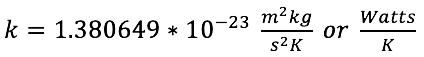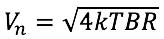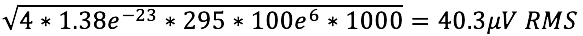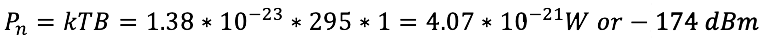|
|### What is Thermal Noise?

March 22, 2023Boltzmann’s constant, k, gives the proportionality of the average kinetic energy of a particle in a system of particles at temperature K (degrees Kelvin). There will exist a statistical distribution of particles with higher or lower energy, but the average over a sufficiently great number of particles is useful for further calculations.

Charges within a resistor move about with thermal kinetic energy. If we consider a leaded resistor, then the axial component of current movement generates a voltage on the leads. This random axial charge movement creates an AC noise voltage on the resistor leads equal to:Where k is Boltzmann’s constant
T is the Kelvin temperature
B is the bandwidth of the AC voltage measurement
R is the resistance of the resistor

The factor of 4 comes from the dimensional degrees of freedom accorded to the charges in the resistor and that only the axial component of movement contributes to output noise. The derivation is left up to the student.

For example, a 1 kΩ resistor will generate:in a 100 MHz bandwidth at 22ºC

The thermal noise in a 50Ω environment at room temperature is -174 dBm in a 1 Hz bandwidth. An RF engineer should memorize this number. This comes from the noise power calculation:See this and more important radio frequency charts and formulas in this comprehensive application note